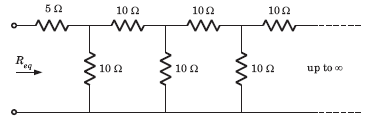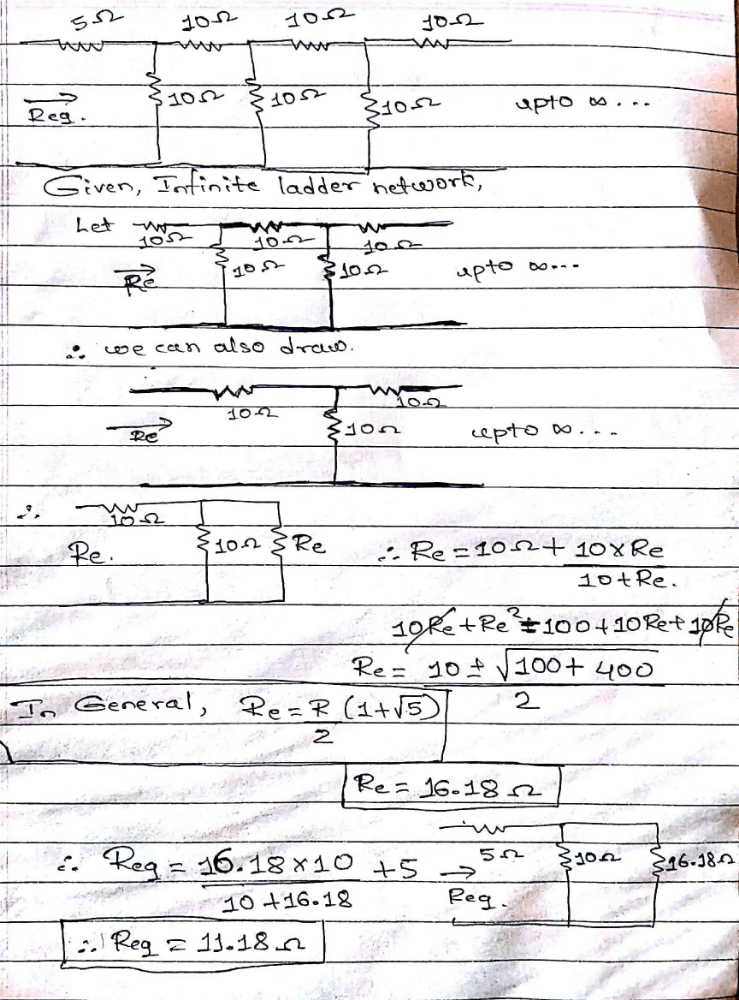Courses

# Req =  ?a)11.86 Ω b)10 Ωc)25 Ω d)11.18 ΩCorrect answer is option 'D'. Can you explain this answer? Related Test: Basic Concepts - MCQ Test

## Electrical Engineering (EE) Question

Shanta B answered Dec 06, 2018Rupesh Handore answered Jul 18, 2018

Suyash Dewangan answered Aug 18, 2018
It is wrong I saw the solution and I found that it has been solved taking 5 ohm resistor....

Balwinder Singh answered Feb 23, 2020
We infer from the given diagram that the same pattern is followed after every 10ohm resistor. The infinite pattern in parallel as a whole is considered as to be Rx.
Thus, Rx = R + (R||Rx)
Solving for Rx, we get Rx = 1.62R, where R=10ohm.
So we have Rx = (1.62ohm)*(10ohm), which gives Rx=16.2ohm
Therefore, Req = 5 + (10||16.2)
=> 5 + [(10*16.2)/(10+16.2)] => Req = 5 + (162/26.2) which gives Req=11.18 ohm.

Krishna Pal Thakur answered Jan 30, 2020
Initial register is of 5 ohm